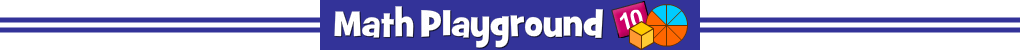Make a trail of factors that multiply to the given product.
Double click the final factor to end the trail.
Number Trails Multiplication - Learning Connections
Essential Skills
Mental Math - practice multiplying numbers

Common Core Connection for Grades 3 and 4
Apply properties of operations as strategies to multiply.
Fluently multiply within 100
Find all factor pairs for a whole number in the range 1-100.
Recognize that a whole number is a multiple of each of its factors.
More Math Games to Play